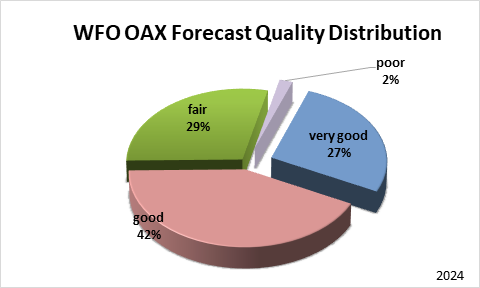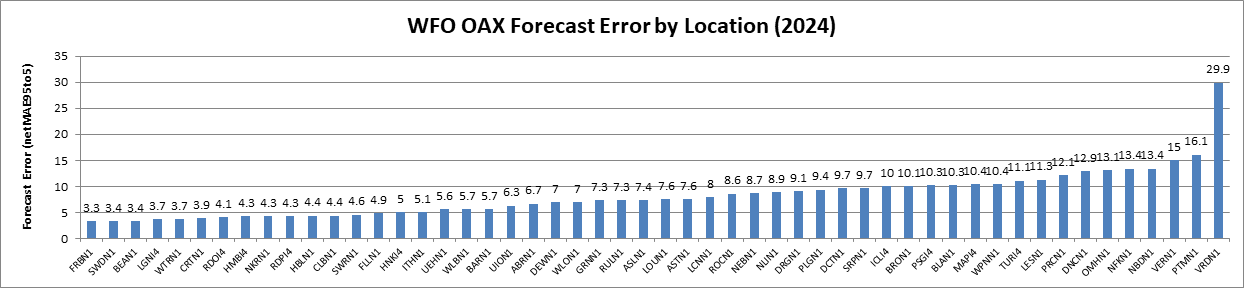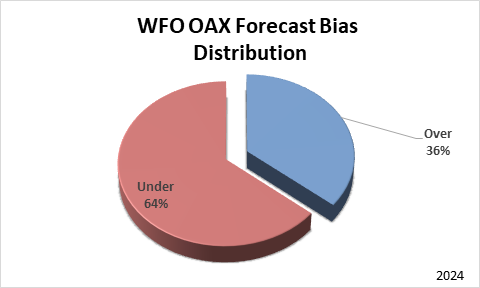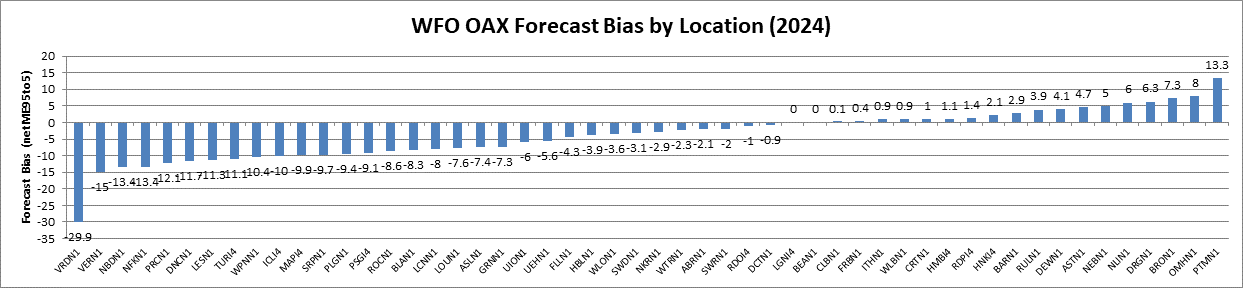Ensemble Streamflow Probabilistic (ESP) Verification

WFO-Omaha, NE (WFO)

### Forecast Quality

Forecast quality is based on the magnitude of the Mean Absolute Error (MAE) also known as forecast error. MAE is the average error of the absolute value between the difference of forecast and observed value for each forecast exceedance probability category (95%, 90%, 75%, 50%, 25%, 10% and 5%) referred to in this discussion as netMAE95to5. The closer the MAE value is to zero the better the forecast.

The Forecast Quality, forecast error based on the netMAE95to5, has five categories, these are:

 very good: ≥ 0% & < 5% good: ≥ 5% & < 10% fair: ≥ 10% & < 20% poor: ≥ 20% & < 30% very poor: ≥ 30%### Forecast Quality by NWS Forecast Point Location Identifier

 very good: ≥ 0% & < 5% good: ≥ 5% & < 10% fair: ≥ 10% & < 20% poor: ≥ 20% & < 30% very poor: ≥ 30%### Forecast Bias

Forecast bias is based on the magnitude of the Mean Error (ME). ME is the average error of between the difference of forecast and observed value for each forecast exceedance probability category (95%, 90%, 75%, 50%, 25%, 10% and 5%) referred to in this discussion as netME95to5.

 - a positive value indicates over forecasting bias - a negative value indicates under forecasting bias - a value of zero indicates no forecast bias### Forecast Bias by NWS Forecast Point Location Identifier

 - a positive value indicates over forecasting bias - a negative value indicates under forecasting bias - a value of zero indicates no forecast bias### Data Analysis Summary by WFO and Forecast Point Location Identifier

For each location designated by an NWS location identifier (NWSLI) in the table below there are two rows of information.

Row 1 contains the NWSLI, the observed exceedance percentage for each forecast exceedance probability (column header PCT), and (sum/cnt) column where sum is the number of occurrences and cnt is the total number of forecasts for each probability category.

Row 2 contains for each forecast exceedance probability whether it was under forecast, over forecast, or spot-on (no bias), followed by the difference of the forecast exceedance probability and observed exceedance percentage. At the end of the row are the net Mean Absolute Error and net Mean Error based on the average of all the categories (95% thru 5%) and a subset of the categories, 90% thru 10%.